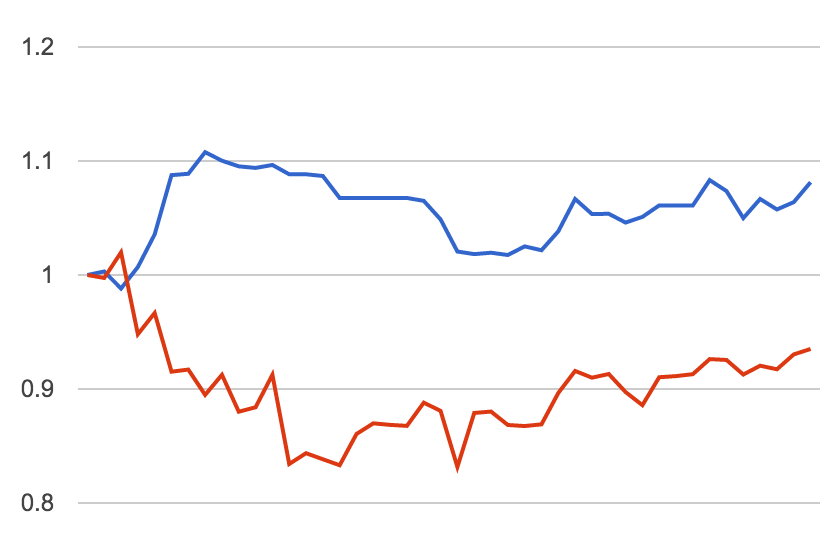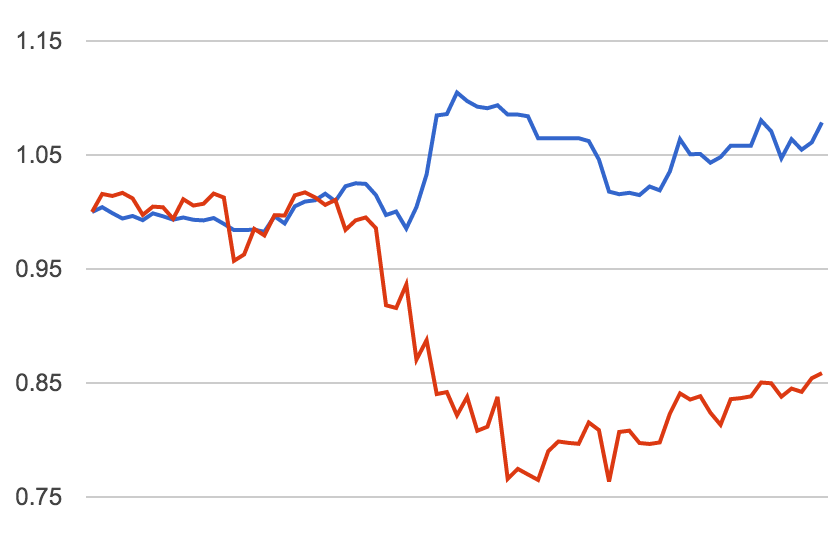### 状态# 分享一个看A股各种估值数据走势的方法

www.jointquant.com

def initialize(context):
g.security = '000826.XSHE'

def handle_data(context, data):
security = g.security
df = get_fundamentals(query(
valuation
).filter(
valuation.code.in_([security])
).order_by(
valuation.market_cap.desc()
))

# df 包含各种数据，把下面的'pe_ratio'改成别的就能画出其他数据的曲线
record(pe_ratio=df['pe_ratio'])


df里有的域是
id
code
pe_ratio
turnover_ratio
pb_ratio
ps_ratio
pcf_ratio
capitalization
market_cap
circulating_cap
circulating_market_cap
day
pe_ratio_lyr

# Java中重载equals方法的大坑(OOP通用)

class A {
int x;

public A(int x) {
this.x = x;
}

@Override
boolean equals(Object o) {
if (o instanceof A) {
return x == (A)(o).x;
} else {
return false;
}
}
}

class B extends A {
int y;

public B(int x, int y) {
super(x);
this.y = y;
}
}


    @Override
boolean equals(Object o) {
if (o instanceof B) {
return x == (B)(o).x && y == (B)(o).y;
} else {
return false;
}
}


1. 自反性。即x.equals(x)为真。
2. 对称性。即x.equals(y) == y.equals(x)
3. 传递性。即x.equals(y) && y.equals(z)，则x.equals(z)

A a = new A(0);
A b = new B(0, 0);
a.equals(b); //true
b.equals(a); //false


A a = new B(0, 0);
A b = new A(0);
A c = new B(0, 1);
a.equals(b); //true
b.equals(c); //true
a.equals(c); //false


    private static final Set <a> luckyA = new HashSet<>()
static {
}

public boolean isLucky(A a) {
return luckyA.contains(a);
}


Wiki上的定义：Subtype Requirement: Let $latex\displaystyle \phi (x)$ be a property provable about objects x of type T. Then $latex\displaystyle \phi (y)$ should be true for objects y of type S where S is a subtype of T.

class B {
A a;
int y;

public asA() {
return a;
}
}


# 2016一季度二级市场投资小结## 痛失老板电器1. 低估值
2. 40元左右大老板增持，员工持股计划，代理商增持，数量还不少
3. 270万台产能生产基地马上15年底完成，进入生产阶段
4. 房地产马上热了起来，整个家电行业增长的预期提升
5. 公司资产负债率很低，现金流充足，想要扩大投资杠杆，随时都可以搞
6. 大宗商品价格大跌，生产成本下降
7. 管理层能力在行业内明显是老大，市场占有率不断提升
8. 看财报，公司营收大幅增长，但是应收账款等却没有怎么涨，可见公司在市场中(可能是对消费者，也可能是对代理商)处于主动地位，货还是挺抢手的。
9. 然而我觉得最可怕的是，2、3两点加起来，有了大幅增加的产能，巴结了代理商，那么要做数据非常好看的报表简直易如反掌了。想象一下只要代理商想，随时可以从老板电器这里拿货，只要代理商拿了货，就可以当成卖出去算进利润了。有了大幅增加的产能，又有了出处，加上第8条货还是挺抢手的，然后代理商大量持股，多拿一点货让自己的持股快速增值，何乐而不为。如果把代理商看成老板电器新的仓库，简直是利润想怎么调怎么调。

## 选股到底该怎么选

1. 资本运作(增减持等)
2. 行业、题材(次新股等也算)
3. 筹码(比如碧水源虽然公司不错，但是谁都知道它好，随便拿个软件看都知道高位套牢的筹码不肯割肉，这样就很难涨)
4. 行业地位(就是竞争力)
5. 经营状况(看自己看财报的功力了)
6. 市值(越小越好)

## 目前观测的股票

• 碧水源(公司来讲别的都很好，就是现金流有点烂，应收账款持续大幅增加，被国家开发银行定增奶了一口，年报还不错，主要看点是定增后的业绩释放(定增前基本要调节利润的))
• 老板电器
• 奥瑞金(做易拉罐的。低估值，实际控制人增持——虽然是迎合救市的，聊胜于无。开始不太想当红牛小弟，扩大投资，举牌永新股份(也是做包装的)，整合包装行业和下游快消品产业链，进军体育行业，目的是为客户提供宣传渠道。感觉布局和视野都还是行的。这些投资都还有理有据，不是在瞎搞。最大的风险是负债率已经很高了，年报60%+，但是公司已经向证监会提了定向增发，还没有答复。等定增弄下来，负债降一降，还是有点意思的)
• 博敏电子(次新股，走势好看)
• 凯龙股份(同上)
• 读者传媒(同上，这个行业很好)

• 奥瑞金
• 博敏电子